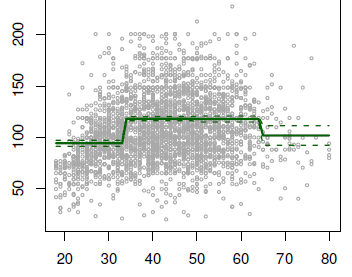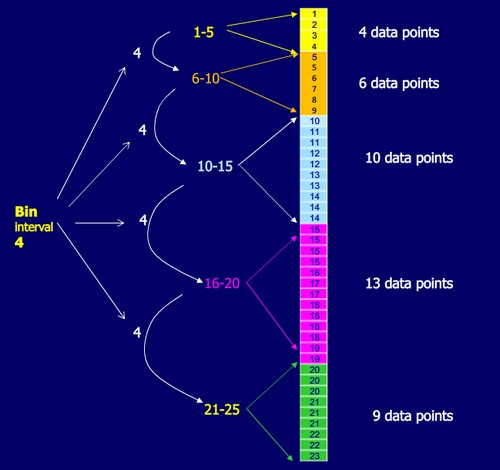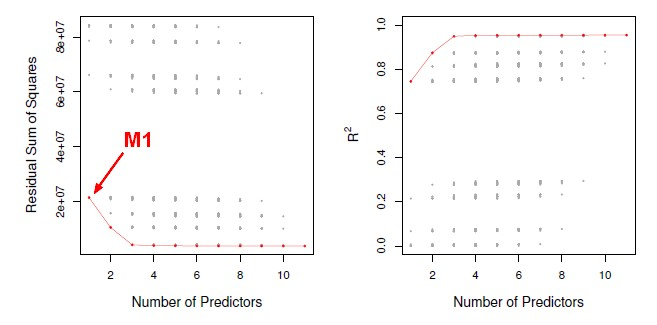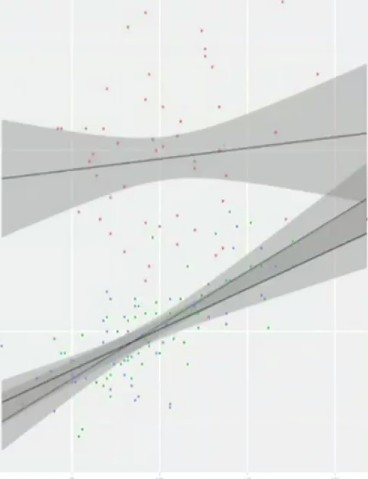# Statistics - Dummy (Coding|Variable) - One-hot-encoding (OHE)

Dummy coding is:

• a classic way to transform nominal into numerical values.
• a system to code categorical predictors in a regression analysis

A system to code categorical predictors in a regression analysis in the context of the general linear model.

We can't put categorical predictors such as character variable, or a string variable into a regression analysis function. We need to make it a numeric variable in some way. That's where dummy coding comes in.

It allows to look at categorical predictors in the same model as continuous predictors and put them together in moderation analyses.

Featurizing via a one-hot-encoding representation lead to a very large feature vector. To reduce the dimensionality of the feature space, feature hashing is generally used.

## Scheme

### Pairwise

Four levels of my (independent|grouping) variable:

• Blue
• Green
• Red
• Yellow
Groups Dummy Code 1
Variable 1
Dummy Code 2
Variable 2
Dummy Code 3
Variable 3
Blue 0 0 0
Green 1 0 0
Red 0 1 0
Yellow 0 0 1

The number of dummy code (dummy variable) is the number of value minus 1. Blue is the reference group and get 0 across the board.

The regression constant in a multiple regression, it's the predicted score on the outcome variable, when all others variables are zero which means that it will be the predicted score for the reference group.

The regression constant will be the mean for Blue because it's the reference group.

In this scheme, you will compare the reference group with the others group. If you want to make a comparison between two groups that are not a reference group, you will have to make one of them the reference group.

These is pairwise comparisons approach and is for this reason generally not used. A one-way ANOVA would be done instead where, you can code a categorical variable in a multiple regression analysis.

### Effects coding

In Effects coding schemes, the regression constant is the predictive score for all individuals, across all groups, not just the predictive score for the reference group.

#### unweighed

Instead of giving O across the board, the reference group get minus one.

Groups Dummy Code 1 Dummy Code 2 Dummy Code 3
Blue -1 -1 -1
Green 1 0 0
Red 0 1 0
Yellow 0 0 1

The codes (variables) are now effects codes instead of dummy codes.

The regression constant will now represent the average across all groups. It will not be exactly the mean for the entire group because it's unweighed as it doesn't take into account the fact that there are different number of individuals into each group.

If you wanted to know the difference between the overall mean and the reference group, you would have to re-code this with a difference reference group.

#### weighted

If you want to get the regression constant to be the exact mean of all individuals, you have to weight the score by the number of individuals in each group.

Groups Dummy Code 1 Dummy Code 2 Dummy Code 3
Blue $- \fracN_{Blue}}N_{Total}$ $- \fracN_{Blue}}N_{Total}$ $- \fracN_{Blue}}N_{Total}$
Green $\fracN_{Green}}N_{Total}$ 0 0
Red 0 $\fracN_{Red}}N_{Total}$ 0
Yellow 0 0 $\fracN_{Yellow}}N_{Total}$

## Example

Dictionary with the mapping (featureId, Value) = NewFeatureId

### Before Mapping

Feature 0: Color

• Red
• Blue
• Purple

Feature 1: Status

• Not started
• In Progress
• Final

### After mapping

• New Feature 0 = (Color (Feature 0), Red)
• New Feature 1 = (Color (Feature 0), Blue)
• New Feature 2 = (Color (Feature 0), Purple)
• New Feature 3 = (Status (Feature 1), Not started)
• New Feature 4 = (Status (Feature 1), In Progress)
• New Feature 5 = (Status (Feature 1), Final)

Recommended PagesData Mining - Step Function (piecewise constants)

Step functions, are another way of fitting non-linearities. (especially popular in epidemiology and biostatistics) Continuous variable are cut into discrete sub-ranges and fit a constant model in...Loss functions (Incorrect predictions penalty)

Loss functions define how to penalize incorrect predictions. The optimization problems associated with various linear classifiers are defined as minimizing the loss on training points (sometime along with...R - (Dummy Code|Categorical Variable) in Regression

Dummy codes in order to handle Categorical Variable With a categorical predictor, dummy codes to represent the nominal variable as numeric using the function C (for contrasts) contrasts...Statistics - (Discretizing|binning) (bin)

Discretization is the process of transforming numeric variables into nominal variables called bin. The created variables are nominal but are ordered (which is a concept that you will not find in true...Statistics - (Factor Variable|Qualitative Predictor)

A factor is a qualitative explanatory variable. Each factor has two or more levels, i.e., different values of the factor. Combinations of factor levels are called treatments. Example: character...Statistics - Model Selection

Model selection is the task of selecting a statistical model from a set of candidate models through the use of criteria's Dimension reduction procedures generates and returns a sequence of possible...Statistics - Moderator Variable (Z) - Moderation

A moderation analysis is a multiple regression analysis. The main reason to run a moderation analysis is to demonstrate how a third variable (Z) changes the correlation between two variables (X and Y)....Time Serie - Seasonality (Cycle detection)

Seasonality is a cycle in time serie. The season is used a discreet regression variable and code it as dummy variables). For instance: 1 if it's the season 0 if it's not the season If you...